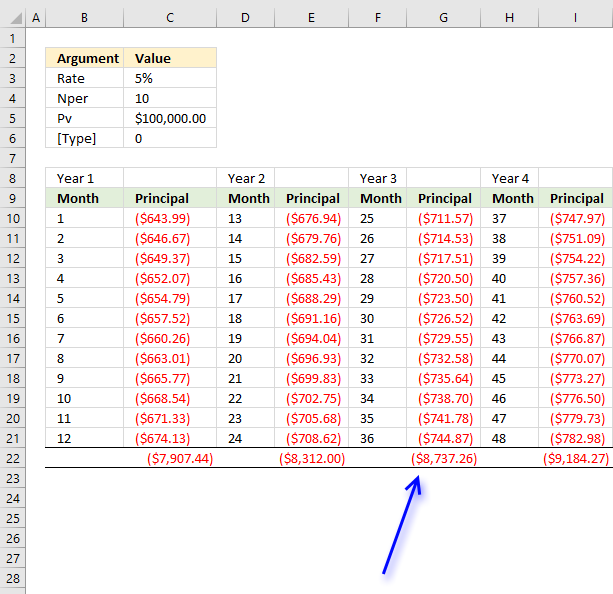Author: Oscar Cronquist Article last updated on September 11, 2018The CUMPRINC function calculates the accumulated principal based on a start and end period on a loan.

The image above shows the CUMPRINC function in cell E3 calculating the accumulated principal for month 25 to 36 for a 10 year loan of 100 000.

Formula in cell E3:

=CUMPRINC(C3/12,C4*12,C5,C6,C7,C8)

### Excel Function Syntax

CUMPRINC(rate, nper, pv, start_period, end_period, type)

### Arguments

 rate Required.  The interest rate. nper Required.  The total number of periods in an annuity. Examples: A monthly payment - 12 Quarterly payment - 4 pv Required.  Present value. start_period Required.  The first period. end_period Required. The last period. type Required.  When payments are due. 0 - End of period. 1 - At the beginning of the period.

The CUMPRINC function returns

• #NUM error value if:
• rate <=0
• nper <=0
• pv<=0
• start_period < 1
• end_period < 1
• start_period > end_period
• type is not 0 (zero) or 1

Use the same unit for rate and nper, the above example uses monthly payments. That is why the interest rate is divided by 12 and nper is multiplied by 12. There are 120 monthly payments in a 10 year period.This image shows the principal for month 25 to 36 and the cumulative principal. The amount in cell G22 is the same as in the top image cell E3.